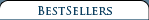3-View Scale Airplane Drawing Books & CDs Aero Albums Books Air Force Legends Air Racing Books Airliner Books Aviation CDs Aviation Century Series Biographicals British Aviation Books Children's Aviation Books Civil Aviation Books A-G Civil Aviation Books H-N Civil Aviation Books O-S Civil Aviation Books T-Z DVDs & Videos Early Aviation Books Golden Age Era Golden Age Of Aviation Series Military Aviation Modeling & R/C Airplane Books Naval Fighters Navy Books Nose Art Books Paul Matt Individual 3-View Drawings Personal Adventures Post World War II Books Soaring, Ultralights & Ballooning Time-Life's Epic Of Flight Series Type Airplane Books A-G Type Airplane Books H-P Type Airplane Books R-Z World War I Aviation Books World War II Aviation Books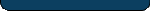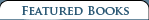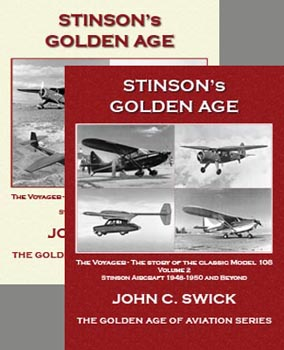Stinson's Golden Age - Vol 1 & Vol 2 \$ 50.00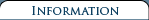Shipping and Returns   Terms and Conditions  Privacy Policy  Contact Us### Ryan Broughams and Their Builders

 Retail Price: \$10.50 By: William Wagner Ryan Broughams and Their Builders is a highly illustrated book about the early Ryan M-1s and Broughams including the most famous of them all, the Spirit of St. Louis, and the 231 similar Ryan aircraft built after LindberghвЂ™s Atlantic crossing. In Ryan Broughams and Their Builders emphasis is given to the following Ryan airplanes: Ryan M-1, M-2 Bluebird, B-1 Brougham, B-3 and B-5.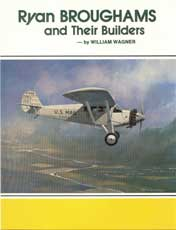A section of the book contains biographies of the company personalities: Don Hall, Franklin Mahoney, Hawley Bowlus and T. Claude Ryan.

Additonaly, detailed information is included for Ryan's businesses: Ryan Airlines, Inc., Ryan Flying Co., B.F. Mahoney Aircraft Corp., Mahoney Ryan Aircraft Corp., Ryan Aircraft Corp., and Ryan Mechanics Monoplane Co. during the 1920s and '30s.

Ryan BROUGHAMS and Their Builders
Book Contents

Chapter HeadingsВ В В В В В В В В В В В В В В В В В В В В В В В В В В В В В В В В В В В В В В В В В В В В В В В В В В В В В В В  Page

Making of a MonoplaneВ В В В В В В В В В В В В В В В В В В В В В В В В В В В В В В В В В В В В В В В В В В В В В В  В В В В В В В В В В В  В В В В В В В В В В В  3
The First OrderВ В В В В В В В В В В В В В В В В В В В В В В В В В В В В В В В В В В В В В В В В В В В В В В В В В В В В В В В В В В В В В В В В В В В В В В В В В В В В В В В В В В  8
Selling the M-1В В В В В В В В В В В В В В В В В В В В В В В В В В В В В В В В В В В В В В В В В В В В В В В В В В В В В В В В В В В В В В В В В В В В В В В В  14
Bluebird and BroughamВ В В В В В В В В В В В В В В В В В В В В В В В В В В В В В В В В В В В В В В В В В В В В В В В В В В В В В В В В В В В В В В В В В В В В В В  19
Debut of the B-1 BroughamВ В В В В В В В В В В В В В В В В В В В В В В В В В В В В В В В В В В В В В В В В В В В В В В В В В В В В В В В В В В В В В В В В  23
Mahoney Takes OverВ В В В В В В В В В В В В В В В В В В В В В В В В В В В В В В В В В В В В В В В В В В В В В В В В В В В В В В В В В В В В В В В В В В В В В В В В В  28
The Special JobsВ В В В В В В В В В В В В В В В В В В В В В В В В В В В В В В В В В В В В В В В В В В В В В В В В В В В В В В В В В В В В В В В В В В В В В В В В В В В В В В В В  35
Enter the St. Louis CrowdВ В В В В В В В В В В В В В В В В В В В В В В В В В В В В В В В В В В В В В В В В В В В В В В В В В В В В В В В В В В В В В В В В В В  44
Now ItвЂ™s Mahoney-RyanВ В В В В В В В В В В В В В В В В В В В В В В В В В В В В В В В В В В В В В В В В В В В В В В В В В В В В В В В В В В В В В В В В В В В В  51
B-3s, B-5s and Doodle BugsВ В В В В В В В В В В В В В В В В В В В В В В В В В В В В В В В В В В В В В В В В В В В В В В В В В В В В В В В В В В В В В В  56
Detroit Aircraft Buys InВ В В В В В В В В В В В В В В В В В В В В В В В В В В В В В В В В В В В В В В В В В В В В В В В В В В В В В В В В В В В  61
B-7 and C-1В В В В В В В В В В В В В В В В В В В В В В В В В В В В В В В В В В В В В В В В В В В В В В В В В В В В В В В В В В В В В В В В В В В В В В В В В В В В В В В В В В В В В В В  65
Replicas of the вЂњSpiritвЂќВ В В В В В В В В В В В В В В В В В В В В В В В В В В В В В В В В В В В В В В В В В В В В В В В В В В В В В В В В В В В В  70
Pilots and PlanesВ В В В В В В В В В В В В В В В В В В В В В В В В В В В В В В В В В В В В В В В В В В В В В В В В В В В В В В В В В В В В В В В В В В В В В В В В В В В В В В В В В  75
Brougham Recognition ChartВ В В В В В В В В В В В В В В В В В В В В В В В В В В В В В В В В В В В В В В В В В В В В В В В В В В В В В В В В В В В В В В  79В В В В В В В В В В В В В В В В В В В
Postscript: Ryan Mechanics Monoplane Co.В В В В В В В В В В В В В В В В В В В В В В В В В В В В В В В В В В В В В В В В  80В В В В В В В В В В В В В В В В В В В В В В В В В В В В В В В
Personalities: Waterhouse, William J.В В В В В В В В В В В В В В В В В В В В В В В В В В В В В В В В В В В В В В В В  83
Personalities: Hall, Donald A.В В В В В В В В В В В В В В В В В В В В В В В В В В В В В В В В В В В В В В В В В В В В В В В В В В В  85
Personalities: Mahoney, B. FranklinВ В В В В В В В В В В В В В В В В В В В В В В В В В В В В В В В В В В В В В В В В В В В В В В В В В В В В В  87
Personalities: Edwards, A. J.В В В В В В В В В В В В В В В В В В В В В В В В В В В В В В В В В В В В В В В В В В В В В В В В В В В В В В В В В В В В В В В В  89
Personalities: Bowlus, William HawleyВ В В В В В В В В В В В В В В В В В В В В В В В В В В В В В В В В В В В В В  91
Personalities: Ryan, T. ClaudeВ В В В В В В В В В В В В В В В В В В В В В В В В В В В В В В В В В В В В В В В В В В В В В В В В В  92
Ryan B-5 Brougham (Scale Drawings)В В В В В В В В В В В В В В В В В В В В В В В В В В В В В В В В В В В В В  94
Performance and SpecificationsВ В В В В В В В В В В В В В В В В В В В В В В В В В В В В В В В В В В В В В В В В В В В В В В В  96

100 pgs,softbound, 8.5 x 11, scale drawings, many photos.

### Ryan Broughams and Their Builders

#### Item Number: 315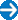Type in the title of book or similar words to help find your item(s).